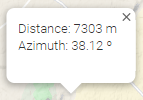﻿ Utilities > Calculate Distances

# CALCULATE DISTANCE

Navegación:  Utilities > Calculate Distances

# CALCULATE DISTANCE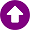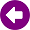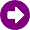This tool calculates the distance and azimuth between two points. You can access this feature by selecting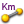on the toolbar. Clicking the viewer the first point of calculation is set. At this time, as you move the cursor over the viewer you can see the distance and azimuth on the status bar that sits just below the viewer. When the second point of calculation is set, the calculation result is showed in an information window.